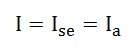# Types of DC Motor

Monday - 26/11/2018 18:48
A Direct Current Motor, DC is named according to the connection of the field winding with the armature. Mainly there are two types of DC Motors. First, one is Separately Excited DC Motor and Self-excited DC Motor.
The self-excited motors are further classified as Shunt wound or shunt motor, Series wound or series motor and Compound wound or compound motor.

The dc motor converts the electrical power into mechanical power is known as dc motor. The construction of the dc motor and generator are same. But the dc motor has the wide range of speed and good speed regulation which in electric traction.

The working principle of the dc motor is based on the principle that the current carrying conductor is placed in the magnetic field and a mechanical force experience by it.

The DC motor is generally used in the location where require protective enclosure, for example, drip-proof, the fireproof, etc. according to the requirements. The detailed description of the various types of the motor is given below.

Separately Excited DC Motor

As the name signifies, the field coils or field windings are energised by a separate DC source as shown in the circuit diagram shown below.Self Excited DC Motor

As the name implies self-excited, hence, in this type of motor, the current in the windings is supplied by the machine or motor itself. Self-excited DC Motor is further divided into shunt wound, and series wound motor. They are explained below in detail.

Shunt Wound Motor

This is the most common types of DC Motor. Here the field winding is connected in parallel with the armature as shown in the figure below.The current, voltage and power equations for a shunt motor are written as follows.

By applying KCL at the junction A in the above figure.

The sum of the incoming currents at A = Sum of the outgoing currents at A.Where,

I is the input line current
Ia is the armature current
Ish is the shunt field current

Equation (1) is the current equation.

The voltage equations are written by using Kirchhoff’s voltage law (KVL) for the field winding circuit.For armature winding circuit the equation will be given asThe power equation is given as

Power input = mechanical power developed + losses in the armature + loss in the field.Multiplying equation (3) by Ia we get the following equations.Where,

VIa is the electrical power supplied to the armature of the motor.

### Series Wound Motor

In the series motor, the field winding is connected in series with the armature winding. The connection diagram is shown below.By applying the KCL in the above figureWhere,

Ise is the series field current

The voltage equation can be obtained by applying KVL in the above figureThe power equation is obtained by multiplying equation (8) by I we getPower input = mechanical power developed + losses in the armature + losses in the fieldComparing the equation (9) and (10), we will get the equation shown below.

### Compound Wound Motor

A DC Motor having both shunt and series field windings is called a Compound Motor. The connection diagram of the compound motor is shown below.

The compound motor is further subdivided as Cumulative Compound Motor and Differential Compound Motor. In cumulative compound motor the flux produced by both the windings is in the same direction, i.e.In differential compound motor, the flux produced by the series field windings is opposite to the flux produced by the shunt field winding, i.e.The positive and negative sign indicates that direction of the flux produced in the field windings.